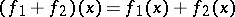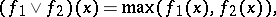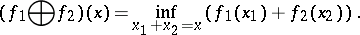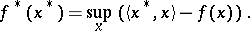# Convex functional

A functional, defined on a convex subset of a linear vector space, the supergraph of which is a convex set. A functionalwhich does not assume the valueon a convex setis convex onif and only if the inequalityis satisfied. If the inequality sign is reversed, the functionalis called concave. Operations which convert a convex functional into a convex functional include addition, multiplication by a positive number, taking the upper boundand the infimal convolutionA convex functional bounded from above in a neighbourhood of some pointis continuous at that point. If a convex functional is finite at some point, it has a (finite or infinite) derivative in any direction at that point. Closed convex functionals (i.e. functionals with convex and closed supergraphs) in locally convex linear topological spaces may be described in a dual way: Such a functional is the least upper bound of the affine functions which it dominates. This duality makes it possible to relate to each convex functional a dual object, the conjugate functionalProperties of convex functionals, operations on such functionals, and the relationship between a convex functional and its conjugate, are studied in convex analysis.